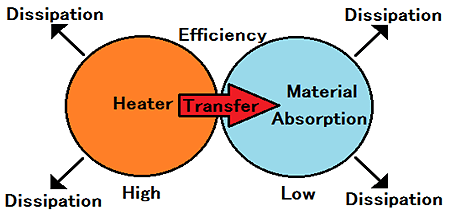MobilePC

PC site display

# 3-3.Heat balance equation of the dryingThe heat balance is the sum of 4 types heat、which is entering heat, exiting heat, generation of heat, and endothermic.

By the law of conservation of energy, the quantity of these

(Quantity of heat change of the system) = (Quantity of heat entering the system) – (Quantity of heat exiting from the system) + (Quantity of heat generation in the system) – (Quantity of heat endothermic in the system)

In the drying process, position energy, kinetic energy, and the work, heat change is the quantity of very small.
They can be ignored, and omitted from consideration element.
In addition, quantity of heat change of the system has is 0 in a general environment such as factories and laboratories.
In the drying process, since it is the one that does not self-heating and ignition, furthermore, the amount of heat is 0.

If it assign a 0

0 = (entering heat) - (exiting heat) + 0 - (endothermic)

When it organize an a formula

(entering heat) = (exiting heat ) + (endothermic)

or

(endothermic) = (entering heat) - (exiting heat )

The quantity of heat entering the system, it is the efficiency of action and the quantity of heat of the heater.
The quantity of heat exiting from the system, it is the quantity of heat radiation and heat conduction from the material.

The endothermic in the system, the quantity of heat used for heating the material, and heat is used for evaporation of water.
The heat used since water evaporates is called “evaporation heat” or “heat of vaporization.”
Drying by heating, is a way to accelerate the drying by continuous supply the heat of vaporization.

### <<Evaporation heat water per 1g >>

 Temp℃ Latent heat 0℃ 20℃ 40℃ 60℃ 80℃ 100℃ 2502J 2454J 2407J 2359J 2309J 2257J

#### << Example Ask for the heating and drying time at water 1g by the 100w-heater. >>

It apply this to a formula on condition of lower.

It need 2454J, to cause the 1g evaporation of water 20 ℃ under atmospheric pressure.

Endothermic quantity inside system = 2454J

Quantity of heat entering the system = Quantity of heater 100w = 100J/s

Efficiency that act on the system = Condition 1:100% Condition 2:50%
Quantity of heat exiting from the system = Condition 1:0 Condition 2:20J/s

Xs = (Quantity of heat endothermic in the system)/(Quantity of heat entering the system- Quantity of heat exiting from the system)

#### Condition 1

Xs = ( 2454J) / (100Jx1.0- 0J)

Xs = 24.54s

Requires heating for about 25 seconds.

#### Condition 2

Xs = ( 2454J) / (100Jx0.5- 20J)
Xs = 81.8s

Requires heating for about 82 seconds.

Even if it heats the same subject with the same heater, if conditions differ, the difference of 3 times or more may appear in heating time.